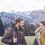# Proof Contest Day 3

$\large{\frac { ab+1 }{ { ( a+b ) }^{ 2 } } +\frac { bc+1 }{ ( b+c ) ^{ 2 } } +\frac { ca+1 }{ { ( c+a ) }^{ 2 } } \ge 3}$

Let $a,b$ and $c$ be positive real numbers such that $a^2+b^2+c^2+(a+b+c)^2 \le 4$. Prove the above inequality.Note by Department 8
5 years, 6 months ago

This discussion board is a place to discuss our Daily Challenges and the math and science related to those challenges. Explanations are more than just a solution — they should explain the steps and thinking strategies that you used to obtain the solution. Comments should further the discussion of math and science.

When posting on Brilliant:

• Use the emojis to react to an explanation, whether you're congratulating a job well done , or just really confused .
• Ask specific questions about the challenge or the steps in somebody's explanation. Well-posed questions can add a lot to the discussion, but posting "I don't understand!" doesn't help anyone.
• Try to contribute something new to the discussion, whether it is an extension, generalization or other idea related to the challenge.

MarkdownAppears as
*italics* or _italics_ italics
**bold** or __bold__ bold
- bulleted- list
• bulleted
• list
1. numbered2. list
1. numbered
2. list
Note: you must add a full line of space before and after lists for them to show up correctly
paragraph 1paragraph 2

paragraph 1

paragraph 2

[example link](https://brilliant.org)example link
> This is a quote
This is a quote
    # I indented these lines
# 4 spaces, and now they show
# up as a code block.

print "hello world"
# I indented these lines
# 4 spaces, and now they show
# up as a code block.

print "hello world"
MathAppears as
Remember to wrap math in $$ ... $$ or $ ... $ to ensure proper formatting.
2 \times 3 $2 \times 3$
2^{34} $2^{34}$
a_{i-1} $a_{i-1}$
\frac{2}{3} $\frac{2}{3}$
\sqrt{2} $\sqrt{2}$
\sum_{i=1}^3 $\sum_{i=1}^3$
\sin \theta $\sin \theta$
\boxed{123} $\boxed{123}$

Sort by:

Here is my solution for the problem:

Since

\begin{aligned} (a+b)^2 + (b+c)^2 + (c+a)^2 &= 2(a^2 + b^2 + c^2 + ab + bc + ca) \\ &= a^2 + b^2 + c^2 + (a + b + c)^2, \end{aligned}

Let

\begin{aligned} \alpha &= b + c \\ \beta &= c + a \\ \gamma &= a + b \end{aligned}

This implies

\begin{aligned} a &= \frac{\beta + \gamma - \alpha}2 \\ b &= \frac{\alpha + \gamma - \beta}2 \\ c &= \frac{\alpha + \beta - \gamma}2 \end{aligned}

With this change of variables, the constraint becomes

$\alpha^2 + \beta^2 + \gamma^2 \le 4,$

while the left side of the inequality we need to prove is now

\begin{aligned} & \frac{\gamma^2 - (\alpha - \beta)^2 + 4}{4\gamma^2} + \frac{\alpha^2 - (\beta - \gamma)^2 + 4}{4\alpha^2} + \frac{\beta^2 - (\gamma - \alpha)^2 + 4}{4\beta^2} \ge \\ & \frac{\gamma^2 - (\alpha - \beta)^2 + \alpha^2 + \beta^2 + \gamma^2}{4\gamma^2} + \frac{\alpha^2 - (\beta - \gamma)^2 + \alpha^2 + \beta^2 + \gamma^2}{4\alpha^2} + \frac{\beta^2 - (\gamma - \alpha)^2 + \alpha^2 + \beta^2 + \gamma^2}{4\beta^2} = \\ & \frac{2\gamma^2 + 2\alpha\beta}{4\gamma^2} + \frac{2\alpha^2 + 2\beta\gamma}{4\alpha^2} + \frac{2\beta^2 + 2\gamma\alpha}{4\beta^2} = \\ & \frac32 + \frac{\alpha\beta}{2\gamma^2} + \frac{\beta\gamma}{2\alpha^2} + \frac{\gamma\alpha}{2\beta^2}. \end{aligned}

Therefore it remains to prove that

$\frac{\alpha\beta}{2\gamma^2} + \frac{\beta\gamma}{2\alpha^2} + \frac{\gamma\alpha}{2\beta^2} \ge \frac32.$.

Which is obviously true by AM-GM.

- 5 years, 6 months ago

Great solution!

- 5 years, 4 months ago

Thank you:-)

- 5 years, 4 months ago

This is 2011 USAMO #1 I believe

- 5 years, 6 months ago

Yes it is.

- 5 years, 6 months ago

Given constraint: a^2+b^2+c^2+ab+bc+ca\leq2 Now, frac{(ab+1)/(a+b)^2}\geq1/2+1/2{(a+b)(b+c)}/(a+b)^2 Cyclic summing over three variables a,b,c And using A.M-G.M we obtain required result

- 5 years, 6 months ago